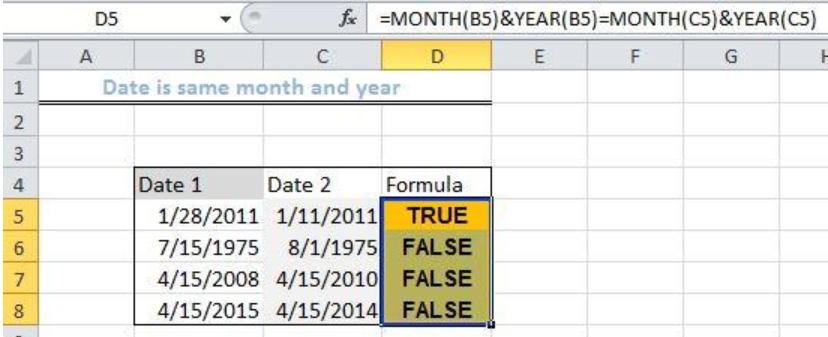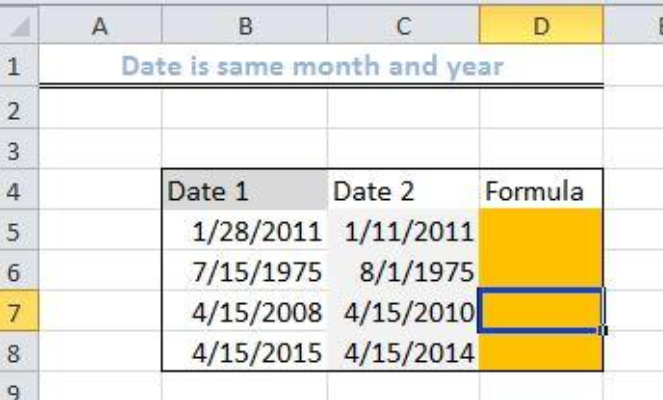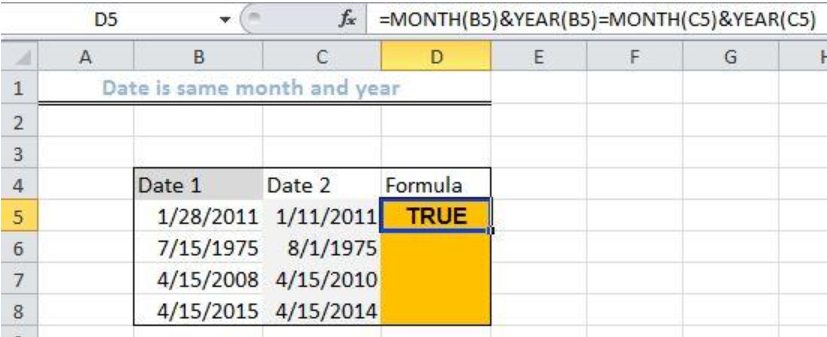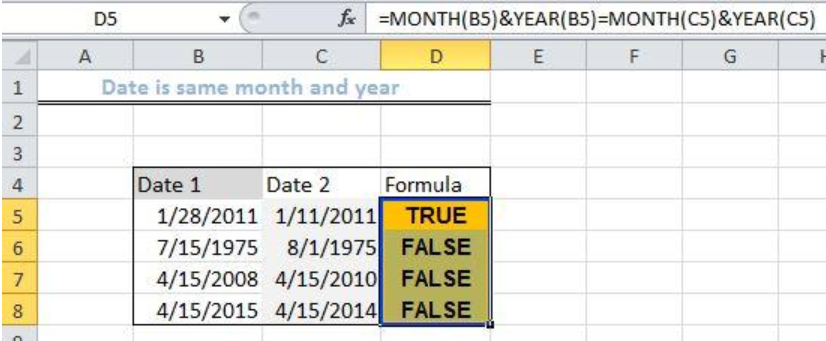Get instant live expert help with Excel or Google Sheets“My Excelchat expert helped me in less than 20 minutes, saving me what would have been 5 hours of work!”

#### Post your problem and you’ll get Expert help in seconds.

Your message must be at least 40 characters
Our professional Expert are available now. Your privacy is guaranteed.

# Date is same month and year

We can use the MONTH and YEAR functions to test if two dates share the same month and year in Excel.  The following steps will walk through the process.Figure 1: Result of Date is the same month and year

## General Formula

`=MONTH(date1)&YEAR(date2)=MONTH(date1)&YEAR(date2)`

## Formula

`=MONTH(B5)&YEAR(B5)=MONTH(C5)&YEAR(C5)`

## Setting up the Data

• We will input our dates in Column B and Column C
• Column B will contain the values for Date 1
• Column C will hold the values for Date 2
• Column D is where we will insert the Formula. It will return TRUE if the date is shared or FALSE, if not.Figure 2: Setting up the Data

## Applying the MONTH and YEAR functions

• We will click on Cell D5
• We will insert the formula below into the cell
`=MONTH(B5)&YEAR(B5)=MONTH(C5)&YEAR(C5)`
• We will press the enter keyFigure 3: Applying the MONTH and YEAR functions

• We will click on Cell D5 again
• We will double click on the fill handle tool (the small plus sign at the bottom right of Cell D5) and drag down to copy the formula into the other cells.Figure 4: Result of Date is the same month and year

## Explanation

`=MONTH(B5)&YEAR(B5)=MONTH(C5)&YEAR(C5)`

With this formula, the month is extracted from the date in Cell B5 as a number. This is represented in the form of a text string. The same will be done with the date in Cell C5. The year and month extracted will be combined. Lastly, the formula will check to see if the dates are equivalent and the formula is solved in the following way:

`=MONTH(B5)&YEAR (B5)=MONTH(C5)&YEAR (C5)`

`=1 & 2011=1 & 2011`

`=“1201”= “1201”`

`=TRUE`

## Instant Connection to an Expert through our Excelchat Service

Most of the time, the problem you will need to solve will be more complex than a simple application of a formula or function. If you want to save hours of research and frustration, try our live Excelchat service! Our Excel Experts are available 24/7 to answer any Excel question you may have. We guarantee a connection within 30 seconds and a customized solution within 20 minutes.

### Did this post not answer your question? Get a solution from connecting with the expert.Another blog reader asked this question today on Excelchat:
Solution examplesUse the Vlookup Function to complete the "employee" column of table 2. Use "job Id" from table 2 as your lookup_value(s) and table 1 as your reference.
Solved by C. H. in 16 minsIf a cell in another sheet is populated I need a vlookup done. If the cell is not populated I need the cell to return blank.
Solved by T. D. in 60 minsI am trying to make a chart that turns a week range red if nothing is entered in the range. If something is entered then I would like it to turn green. Please Help
Solved by E. U. in 43 minsI need a check box to show/hide an answer of an if function
Solved by Z. U. in 23 minsI need a formula to compare the data in two columns and then export the mismatched data in the 3rd column
Solved by S. Q. in 20 mins## Subscribe to Excelchat.coAnother blog reader asked this question today on Excelchat: